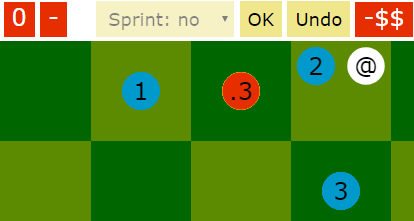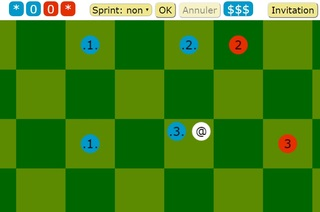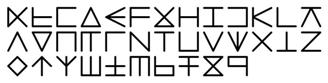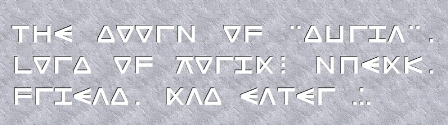# Lucas Borboleta

jeudi 14 juin 2018

## BALTEK game version 1.1

⚽🏃 BALTEK is a turn-based board game, inspired from football.

This release provides a new style for the pages.dimanche 27 mai 2018

## BALTEK game version 1.0

🏃BALTEK is a turn-based board game, inspired from football.

The release v1.0 provides two modes:

• Two close players sharing the same web browsers.
• Two remote players using synchronized web browsers.

The next step will be the implementation of an AI.samedi 26 novembre 2016

## Dutrilin vectorial font published on FontSpace

The runic style font "Dutrilin" has been publish on the FontSpace web site under this URL

On FontSpace, one can "browse a collection of over 30,000 free fonts shared by designers around the world".

Dutrilin provides runic style font. Its letters, in two or three straight lines, result from rotations, reflections and mutations of the Latin and Greek alphabets. Pronounce “Doo-Tri-Lyn”. Dutrilin targets sci-fi and fantasy contexts.

Dutrilin is licensed as Creative Commons (by-sa) Attribution Share Alike.dimanche 1 décembre 2013

## Inner Product Space Characterization by Isometry Group over Unity Sphere

Why “power 2” in the Pythagorean theorem ?

Let us assume a geometry over the set E considered as 1) a vector space over the real field ; 2) with finite dimensions ; 3) equiped with a norm.

Which additional axioms have to be added in order to conclude that E is euclidean, or equivalently, that its norm is deriving from an inner product. Such additionnal axioms are searched in topology and in group formulations, as opposed to calculational ones, like the “polarisation identity”.

The attached document gathers theorems that proof the following axiom solution: 4) if a group G of isometries allows transportation between any pair of points of the unity sphere of E, then E is euclidean.

Borboleta_2013_Inner-Product-Space-Characterization-by-Isometric-Group-over-Unity-Sphere.pdfdimanche 24 novembre 2013

## Return on the 1932 proof of the Auerbach Theorem about Bounded Linear Groups

In 1932, Auerbach proved that, in any finite vector normed space, each bounded linear group left invariant a quadratic and positive form. This work revisits his proof with modern notation, and aims at paying attention to weaknesses: Borboleta_2013_Return-on-Auerbach-Theorem-for-bounded-linear-group.pdf.

dimanche 14 juillet 2013

## MathSAN: Maths with Symbolic Ascii Notation

How to write and work electronic mathematical text without WYSIWYG software or LaTeX?  This memo aims at proposing  MathSAN, an acronym for Mathematics with Symbolic Ascii Notation, focusing on mathematical symbolic or semantic rather than typographic rendering: PDF memo, text memo.

Samples:
```A .i B := {x: x .e A and x .e B }
sum[ i .e NAT ; 1<= i <= n ] = n*(n+1)/2
der[ x**3*y**2 ; x.2 ; y ] = 12*x*y
int[ x**2*dx ; a <= x <= b] = (b**3 - a**3)/3

```

dimanche 14 octobre 2012

## Return on the 1932 proof of the Mazur-Ulam Theorem

In 1932, Mazur and Ulam proved that any isometry f of a normed space E , with f(0)=0, is necessarily a linear transformation. The following article revisits that theorem and its proof: Borboleta_2012_Return-on-the-1932-proof-of-the-Mazur-Ulam-Theorem.pdf

dimanche 23 septembre 2012

## Homework on Jordan-Neumann Inner Product Space Characterization

Here is an homework that revisits  (in the simplest case of real vector space) the proof of 1935 by Jordan and von Neumann, that a vector normed space satisfying the parallelogram law can be equiped with an inner productBorboleta_2012_Homework-on-Jordan-Neumann-Inner-Product-Spaces-Characterization.pdf.

In this proof, the triangular inequality proprety of the norm is invoked for a continuity argument, but otherwise not used.

dimanche 15 juillet 2012

## The group of linear isometries in R2 is discrete and finite group any p-norm, except for p=2!

The euclidean norm is special among the p-norms. Indeed, let us focus on the plan R2. The set of linear isometries in R2, for any p-norm, always composes a group. But for any p-norm, except for p=2, such group is discrete and finite; basiclly it is generated by median and diagonal reflections. Only for p=2, such group of linear isometries is continous. This is showed in the article Borboleta_2012_Linear-Isometries-in-R2-for-P-Norms.